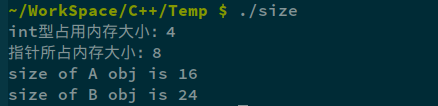# sizeof计算含有虚函数的类对象的大小

2016/11/02 21:07

## 看下面的程序，输出的结果是多少？

（在64位Linux下int占4个字节，指针占8个字节）

#include <iostream>
using namespace std;

class A
{
public:
A(int x) : a(x)
{ }
virtual void print()
{
cout << "A" << endl;
}
private:
int a;
};

class B : public A
{
public:
B(int x) : A(x-1), b(x)
{ }
virtual void print()
{
cout << "B" << endl;
}
private:
int b;
int d;
};

int main()
{
int *p;
cout << "int型占用内存：" << sizeof(int) << endl;
cout << "指针所占内存：" << sizeof(p) << endl;
A a(1);
cout << "size of A obj is " << sizeof(a) << endl;
B b(2);
cout << "size of B obj is " << sizeof(b) << endl;
}• 对于A类来说，由于它含有虚函数，因此占用的内存除了一个整型变量外，还包括一个隐含的虚表指针成员，一共占16个字节（考虑内存对齐：8+4+4
• 对于B类来说，比A类多一个整型成员，因而多四个字节：8+4+4+4+4=24### 作者的其它热门文章

0
0 收藏

0 评论
0 收藏
0